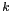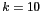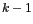DATA MINING
Desktop Survival Guide
by Graham Williams# Cross Validation

In R see the errorest() function in the ipred package.

Cross validation is a method for estimating the true error of a model. When a model is built from training data, the error on the training data is a rather optimistic estimate of the error rates the model will achieve on unseen data. The aim of building a model is usually to apply the model to new, unseen data--we expect the model to generalise to data other than the training data on which it was built. Thus, we would like to have some method for better approximating the error that might occur in general. Cross validation provides such a method.

Cross validation is also used to evaluate a model in deciding which algorithm to deploy for learning, when choosing from amongst a number of learning algorithms. It can also provide a guide as to the effect of parameter tuning in building a model from a specific algorithm.

Test sample cross-validation is often a preferred method when there is plenty of data available. A model is built from a training set and its predictive accuracy is measured by applying the model a test set. A good rule of thumb is that a dataset is partitioned into a training set (66%) and a test set (33%).

To measure error rates you might build multiple models with the one algorithm, using variations of the same training data for each model. The average performance is then the measure of how well this algorithm works in building models from the data.

The basic idea is to use, say, 90% of the dataset to build a model. The data that was removed (the 10%) is then used to test the performance of the model on ``new'' data (usually by calculating the mean squared error). This simplest of cross validation approaches is referred to as the holdout method.

For the holdout method the two datasets are referred to as the training set and the test set. With just a single evaluation though there can be a high variance since the evaluation is dependent on the data points which happen to end up in the training set and the test set. Different partitions might lead to different results.

A solution to this problem is to have multiple subsets, and each time build the model based on all but one of these subsets. This is repeated for all possible combinations and the result is reported as the average error over all models.

This approach is referred to as k-fold cross validation whereis the number of subsets (and also will be the number of models built). Research indicates that there is little to gain by using more than 10 partitions, so usually. That is, the available data is partitioned into 10 subsets (each contains 10% of the available data). The holdout method is then replicatedtimes, each time combining(i.e., 9) subsets to form the training set (consisting of 90% of the original data), and the remaining subset (10%) is the test set.

Some prefer test sample cross-validation where a classification tree is built from a training dataset and the predictive accuracy is tested by predicting on a test dataset. The costs for the test dataset are compared to those for training dataset (cost is the proportion of misclassified cases when priors are estimated and misclassification costs are equal). Poor cross-validation when test costs are hight.

k-fold cross-validation is useful when no test dataset is available (e.g., the available dataset is too small). k is the number of nearly equal sized random subsamples. Build model k times leaving out one of the subsamples each time. The remaining subsample is used as a test dataset for cross-validation. The cross validation costs computed for each of the k test samples are then averaged to give the k-fold estimate of the cross validation costs.